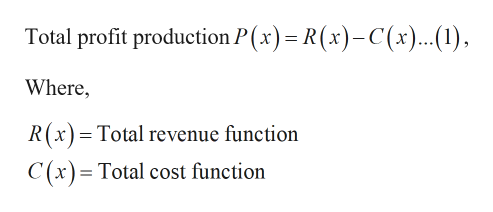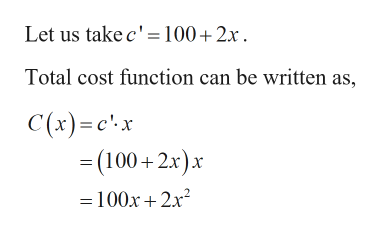# The weekly demand function for x units of a product sold by only one fir, is p=300-1/2x, and the average cost of production and sale is bar c= 100+2x dollars. find the x value wich maximizes profit; also find the price which maximises that profit.

Question
6 views

The weekly demand function for x units of a product sold by only one fir, is p=300-1/2x, and the average cost of production and sale is bar c= 100+2x dollars. find the x value wich maximizes profit; also find the price which maximises that profit.

check_circle

Step 1

Given:

Step 2

Calculation:help_outlineImage TranscriptioncloseTotal profit production P(x) R(x)-C(x)..(1), Where, R(x) Total revenue function C (x)= Total cost function fullscreen
Step 3help_outlineImage TranscriptioncloseLet us take c' 100+2x Total cost function can be written as, C(x)c'x =(100+2x)x = 100x2x2 fullscreen

### Want to see the full answer?

See Solution

#### Want to see this answer and more?

Solutions are written by subject experts who are available 24/7. Questions are typically answered within 1 hour.*

See Solution
*Response times may vary by subject and question.
Tagged in

### Other